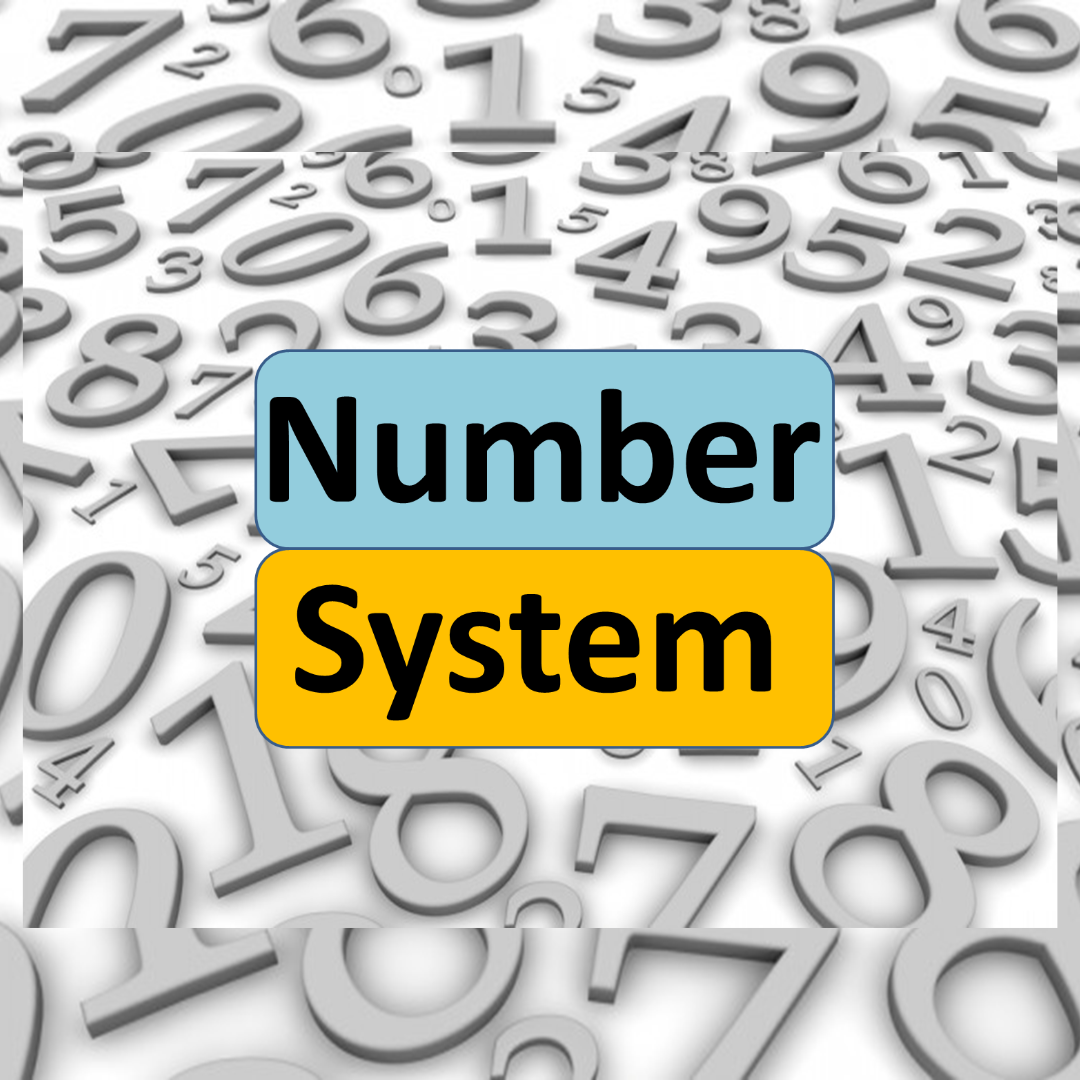# Number System

Number System is considered one of the most important topics under Quantitative Aptitude section in all the Competitive Examinations. The reason being a wide variety of questions are asked from this topic. Enroll for this ‘Number System’ course and prepare this topic completely. The Course covers all the important concepts involved in Number System, Tips and Tricks to solve all types of Questions, Practice and Test Quizzes, Time saving Tricks and many more.

₹ 20.00
₹ 20.00
Last Update 05/22/2021 08:49 Hours 7
Key Features :
• Course Validity 1 Year
• Short tricks
• Strong -Weak Areas Analysis
• Bi-lingual
• Latest Syllabus 2022-23
• Speed, Accuracy Boosters
• Revision Class
• 200+ Practice Questions

• Introduction - 0
• Free preview
• Divisibility Rules in Number System - 0
• Important Divisibility Rules from 1 to 13 - Tricks
• Polynomial Remainder Theorem - 0
• Polynomial Remainder Theorem - Algebraic Formulas
• Calculating Remainder Questions
• Practice Questions on Remainder Theorem
• Arithmetic and Geometric Series - 0
• Arithmetic & Geometric Sequence - Definition, Formulae, Examples
• Arranging Fractions - 0
• Arranging Fractions - Smallest-Largest/ Ascending-Descending
• Questions Session on Smallest Largest Fraction
• Practice Questions on Finding Smallest/Largest Fraction
• Number System - Arithmetic Operations and Fractions of Numbers - 0
• Operations based Questions - Division Multiplication Addition Subtraction
• Question Session on Fractions of Numbers
• Operations based Practice Questions - Division & Multiplication
• Operations based Practice Questions - Addition & Subtraction
• Practice Questions based on Fraction of Numbers
• HCF - LCM and Unit Digit - 0
• Problems on HCF and LCM - Formulas & Tricks
• Practice Questions - LCM and HCF based Problems
• How to find Unit Digit in different Conditions
• Practice Questions on Finding Unit Digit
• Number System - Natural, Odd, Even, Prime Numbers - 0
• Questions based on Sum of first n Even/Odd/Prime/Natural Numbers
• Practice Questions on Consecutive, Odd & Even Numbers
• Practice Questions on Natural & Prime Numbers
• Number System - Miscellaneous - 0
• Important Questions on Different Conditions
• Number System - Practice Test Series - 0
• Number System Practice Test 1
• Number System Practice Test 2
• Number System Practice Test 3
• Number System - Special Questions (Solved) - 0
• Special Question 1
• Special Question 2
• Special Question 3
• Special Question 4
• Special Question 5
• Special Question 6
• Special Question 7
• Special Question 8
• Special Question 9
• Special Question 10
• Special Question 11
• Special Question 12
• Special Question 13
• Special Question 14
• Special Question 15
• Special Question 16
• Special Question 17
• Number System - Practice Quizzes - 0
• Practice Quiz 1 - Easy
• Practice Quiz 2 - Moderate
• Practice Quiz 3 - Advanced
• Number System - Short Tricks - 0
• Divisibility Short Tricks
• Short Tricks - Divide by 9 with Remainder
• Short Tricks - Multiplication by 99, 999, 999
• Short Tricks - Multiply Just Above 10
• Short Tricks - Number Tree
• Introduction
• Divisibility Rules in Number System
• Polynomial Remainder Theorem
• Arithmetic and Geometric Series
• Arranging Fractions
• Number System - Arithmetic Operations and Fractions of Numbers
• HCF - LCM and Unit Digit
• Number System - Natural, Odd, Even, Prime Numbers
• Number System - Miscellaneous
• Number System - Practice Test Series
• Number System - Special Questions (Solved)
• Number System - Practice Quizzes
• Number System - Short Tricks